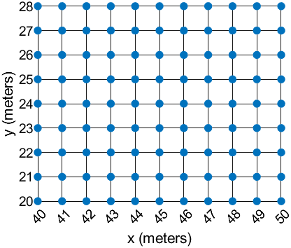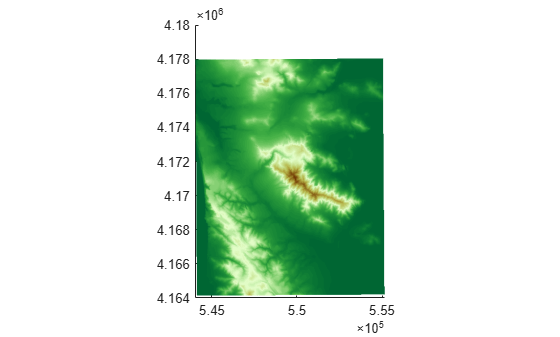# MapPostingsReference

Reference raster postings to map coordinates

## Description

A map postings raster reference object contains information that describes the relationship between a planar map coordinate system and an intrinsic coordinate system that is anchored to the columns and rows of a 2-D spatially referenced grid of point samples (or postings).

Typically, the raster is sampled regularly in the planar world x- and world y-coordinates of the map system, such that the intrinsic x and world xaxes align and the intrinsic y and world y axes align. When this is true, the relationship between the two systems is rectilinear. More generally, and much more rarely, their relationship is affine. The affine relationship allows for a possible rotation (and skew). In either case, rectilinear or affine, the sample spacing from row to row need not equal the sample spacing from column to column.

This image illustrates a 9-by-11 planar raster of postings. The postings are spaced 1 meter apart in x and y, cover x values in the range [40,50] in meters, and cover y values in the range [20,28] in meters. The boundary of the raster of postings is a rectangle with edges that pass through the outermost postings.To reference a raster of cells to planar map coordinates, use a `MapCellsReference` object instead.

## Creation

You can use these functions to create `MapPostingsReference` objects:

## Properties

expand all

Limits of raster in world x-coordinates, specified as a two-element row vector of the form `[xMin xMax]`.

The value of the `ProjectedCRS` property determines the length units for the raster. This code shows how to find the length units for a raster associated with the map postings reference object `R`.

`R.ProjectedCRS.LengthUnit`

Example: `[207000 209000]`

Data Types: `double`

Limits of raster in world y-coordinates, specified as a two-element row vector of the form `[yMin yMax]`.

The value of the `ProjectedCRS` property determines the length units for the raster. This code shows how to find the length units for a raster associated with the map postings reference object `R`.

`R.ProjectedCRS.LengthUnit`

Example: `[911000 913000]`

Data Types: `double`

Number of rows and columns of the raster or image associated with the referencing object, specified as a two-element vector, [m n], where m represents the number of rows and n the number of columns. For convenience, you can assign a size vector having more than two elements. This enables assignments like `R.RasterSize = size(RGB)`, where `RGB` is m-by-n-by-3. In cases like this, the object stores only the first two elements of the size vector and ignores the higher (nonspatial) dimensions. m and n must be positive in all cases and must be 2 or greater.

Example: `[200 300]`

Data Types: `double`

Geometric nature of the raster, specified as `'postings'`. The value `'postings'` indicates that the raster comprises a grid of sample points, where rows or columns of samples run along the edge of the grid. For an m-by-n raster, points with an intrinsic x-coordinate of 1 or n or an intrinsic y-coordinate of 1 or m fall right on an edge (or corner) of the raster.

Cannot be set.

Data Types: `char`

Edge from which column indexing starts, specified as `'south'` or `'north'`.

Example: `ColumnsStartFrom: 'south'`

Data Types: `char`

Edge from which row indexing starts, specified as `'west'` or `'east'`.

Example: `RowsStartFrom: 'east'`

Data Types: `char`

East-west distance between adjacent postings, specified as a positive numeric scalar. The value is constant throughout the raster.

Example: `2.5`

Data Types: `double`

North-south distance between adjacent postings, specified as a positive numeric scalar. The value is constant throughout the raster.

Example: `2.5`

Data Types: `double`

Extent of the full raster or image as measured in the world system in a direction parallel to its rows, specified as a positive numeric scalar. In the case of a rectilinear geometry, which is most typical, this is the horizontal direction (east-west).

Data Types: `double`

Extent of the full raster or image as measured in the world system in a direction parallel to its columns. In the case of a rectilinear geometry, which is most typical, this is the vertical direction (north-south).

Data Types: `double`

Raster limits in intrinsic x-coordinates, specified as a two-element row vector of positive integers, ```[xMin xMax]```. For an m-by-n raster, `XIntrinsicLimits` equals [1 m], because the `RasterInterpretation` is `'postings'`.

Example: `[2 4]`

Data Types: `double`

Raster limits in intrinsic y-coordinates, specified as a two-element row vector of positive integers, ```[yMin yMax]```. For an m-by-n raster with `RasterInterpretation` equal to `'postings'`, `YIntrinsicLimits` equals [1 m].

Data Types: `double`

Type of geometric relationship between the intrinsic coordinate system and the world coordinate system, specified as either `'rectilinear'` or `'affine'`. Its value is `'rectilinear'` when world x depends only on intrinsic x and vice versa, and world y depends only on intrinsic y and vice versa. When the value is `'rectilinear'`, the image displays without rotation in the world system, although it might be flipped. Otherwise, the value is `'affine'`.

Data Types: `char`

Type of coordinate system to which the image or raster is referenced, specified as `'planar'`.

Data Types: `char`

Projected coordinate reference system (CRS), specified as a `projcrs` object. A projected CRS consists of a geographic CRS and several parameters that are used to transform coordinates to and from the geographic CRS.

The value of `ProjectedCRS` determines the length units for the raster. To find the length units, query the `LengthUnit` property of the `projcrs` object.

## Object Functions

 `contains` Determine if geographic or map raster contains points `firstCornerX` Return world x-coordinate of map raster index (1,1) `firstCornerY` Return world y-coordinate of map raster index (1,1) `intrinsicToWorld` Transform intrinsic to planar world coordinates `sizesMatch` Determine if geographic or map raster object and image or raster are size-compatible `worldFileMatrix` Return world file parameters for transformation `worldGrid` World coordinates of raster elements `worldToDiscrete` Transform planar world to discrete coordinates `worldToIntrinsic` Transform planar world to intrinsic coordinates

## Examples

collapse all

Import elevation data as an array and a `MapPostingsReference` object. Then, display the data on a map.

For this example, specify the file to import by extracting a GZ file into a temporary directory. Then, set the name of the first extracted file to a variable.

```filenames = gunzip('sanfranciscos.dem.gz',tempdir); filename1 = filenames{1};```

Import the data by using the `readgeoraster` function. Prepare the data for plotting by specifying the output type as `'double'` and by replacing missing data with `NaN` values.

```[Z,R] = readgeoraster(filename1,'OutputType','double'); info = georasterinfo(filename1); Z = standardizeMissing(Z,info.MissingDataIndicator);```

View the properties of the `MapPostingsReference` object.

`R`
```R = MapPostingsReference with properties: XWorldLimits: [544050 555150] YWorldLimits: [4164120 4178070] RasterSize: [466 371] RasterInterpretation: 'postings' ColumnsStartFrom: 'north' RowsStartFrom: 'west' SampleSpacingInWorldX: 30 SampleSpacingInWorldY: 30 RasterExtentInWorldX: 11100 RasterExtentInWorldY: 13950 XIntrinsicLimits: [1 371] YIntrinsicLimits: [1 466] TransformationType: 'rectilinear' CoordinateSystemType: 'planar' ProjectedCRS: [1x1 projcrs] ```

Display the elevation data as a surface on a map. Apply a colormap that is appropriate for elevation data.

```mapshow(Z,R,'DisplayType','surface') demcmap(Z)```expand all

Introduced in R2013b

## SupportGet trial now